### Statistical Modeling and Identification

#### Course Description

The role of statistical modeling and identification in modern science (an example). Descriptive statistics and data visualization (histogram, scatter graph, box plots). Goodness of fit tests. Description and evaluation of statistical properties of an estimate: biasness, efficiency, consistency, sufficiency and completeness (Neyman-Fisher factorization theorem, Rao-Blackwell-Lehmann-Scheffe theorem). Methods for finding the estimate: minimum variance unbiased criterion (MVU estimate), maximum likelihood estimation (ML) and CR lower bound, BLUE, method of moments, Bayesian methods. Departures from assumptions (errors-in-variables, instrumental variables). Comparison and selection of methods. Model evaluation and selection (F-test and normal-plot, Akaike information criterion, Mallows Cp). Regression and correlation. Factor analysis and principal component analysis (PCA; scree plot).

#### Literature

Steven M. Kay (1998.), Fundamentals of Statistical Signal Processing: Estimation Theory, Prentice Hall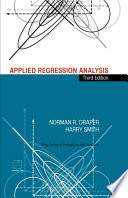Norman R. Draper, Harry Smith (1998.), Applied Regression Analysis, John Wiley & Sons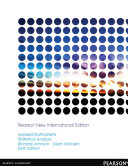Richard A. Johnson, Dean W. Wichern (2013.), Applied Multivariate Statistical Analysis: Pearson New International Edition, Pearson Higher Ed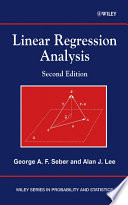George A. F. Seber, Alan J. Lee (2012.), Linear Regression Analysis, John Wiley & Sons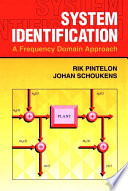Rik Pintelon, Johan Schoukens (2004.), System Identification, John Wiley & Sons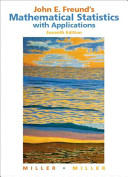Irwin Miller, Marylees Miller (2004.), John E. Freund's Mathematical Statistics with Applications, 7th ed., Prentice Hall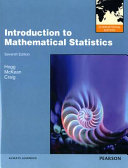Robert V. Hogg, Joseph W. McKean, Allen Thornton Craig (2013.), Introduction to Mathematical Statistics, Pearson Educacion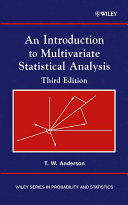Theodore W. Anderson (2003.), An Introduction to Multivariate Statistical Analysis, Wiley-Interscience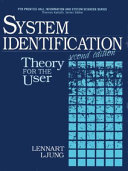Lennart Ljung (1999.), System Identification, Prentice Hall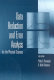Philip Bevington, D. Keith Robinson (2003.), Data Reduction and Error Analysis for the Physical Sciences, McGraw-Hill Science Engineering

#### General

ID 154763
Summer semester
6 ECTS
L1 English Level
L1 e-Learning